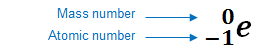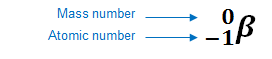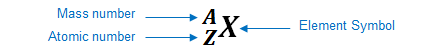# Problem: Write balanced nuclear equations for the following:(a) Formation of 24195Am through β− decay

###### FREE Expert Solution

To write and balance the nuclear equation:

• balance mass number and atomic number of reactants and products

Recall that:

• beta decay or beta emission occurs when an unstable nucleus ejects a beta particle to create a new element
• a beta particle has no atomic mass and is represented by an electronorThe element can be represented as:89% (91 ratings)###### Problem Details

Write balanced nuclear equations for the following:

(a) Formation of 24195Am through β− decay